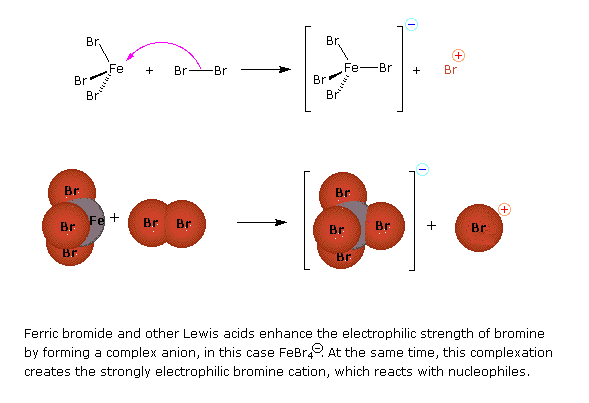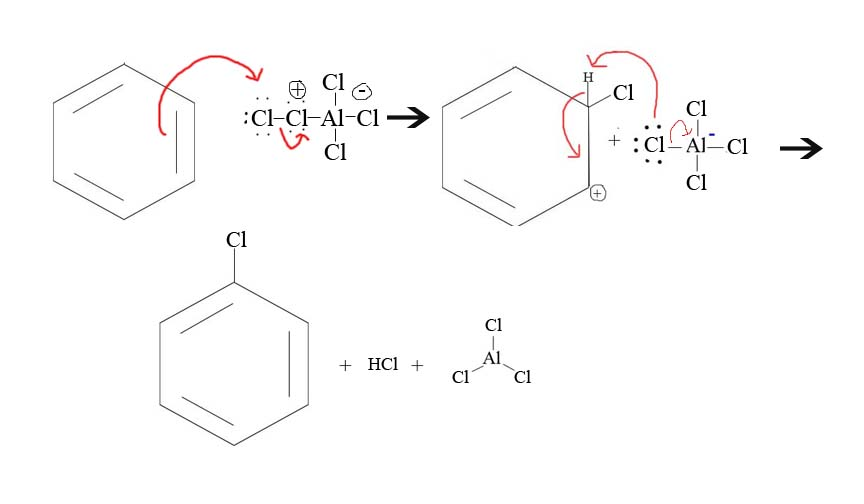# 17.2: Halogenation of Benzene (an EAS Reaction)

$$\newcommand{\vecs}{\overset { \rightharpoonup} {\mathbf{#1}} }$$ $$\newcommand{\vecd}{\overset{-\!-\!\rightharpoonup}{\vphantom{a}\smash {#1}}}$$$$\newcommand{\id}{\mathrm{id}}$$ $$\newcommand{\Span}{\mathrm{span}}$$ $$\newcommand{\kernel}{\mathrm{null}\,}$$ $$\newcommand{\range}{\mathrm{range}\,}$$ $$\newcommand{\RealPart}{\mathrm{Re}}$$ $$\newcommand{\ImaginaryPart}{\mathrm{Im}}$$ $$\newcommand{\Argument}{\mathrm{Arg}}$$ $$\newcommand{\norm}{\| #1 \|}$$ $$\newcommand{\inner}{\langle #1, #2 \rangle}$$ $$\newcommand{\Span}{\mathrm{span}}$$ $$\newcommand{\id}{\mathrm{id}}$$ $$\newcommand{\Span}{\mathrm{span}}$$ $$\newcommand{\kernel}{\mathrm{null}\,}$$ $$\newcommand{\range}{\mathrm{range}\,}$$ $$\newcommand{\RealPart}{\mathrm{Re}}$$ $$\newcommand{\ImaginaryPart}{\mathrm{Im}}$$ $$\newcommand{\Argument}{\mathrm{Arg}}$$ $$\newcommand{\norm}{\| #1 \|}$$ $$\newcommand{\inner}{\langle #1, #2 \rangle}$$ $$\newcommand{\Span}{\mathrm{span}}$$$$\newcommand{\AA}{\unicode[.8,0]{x212B}}$$

## A Mechanism for Halogenation of Benzene

A three-step mechanism is common for many electrophilic aromatic substitution reactions. In the first step, a strong electrophile is created to entice the pi electrons of the aromatic ring to react. In the second, slow or rate-determining step a pair of pi electrons from the benzene form a sigma-bond with the electrophile generating a positively charged sigma complex (the benzenonium intermediate for halogenation). In the third, fast step, a proton is removed from the sigma complex producing a halogenated benzene ring. The steps are illustrated below.

Step 1: Formation of a strong electrophile, in this case an electrophilic bromine cation.Step 2: Pi electrons of benzene react with the bromine cation to form the sigma comoplex, resonance stabilized benzenonium intermediate. This step is the rate determining step.

Step 3: Deprotonation of the benzenonium intermediate (sigma complex) to restore aromaticity.The reaction energy diagram below shows Steps 2 and 3 of the mechanism since these steps involve benzene.Exercises

1. What reagents would you need to get the given product?What reagents would you need to gete given product2. What product would result from the given reagents?3. What is the major product given the reagents below?4. Draw the formatin of Cl+ from AlCl3 and Cl2

5. Draw the mechanism of the reaction between Cl+ and a benzene.

1. Cl2 and AlCl3 or Cl2 and FeCl3

2. No Reaction

3.4.5.